Test: Operational Amplifier

# Test: Operational Amplifier - Electronics and Communication Engineering (ECE)

Test Description

## 10 Questions MCQ Test Topicwise Question Bank for Electronics Engineering - Test: Operational Amplifier

Test: Operational Amplifier for Electronics and Communication Engineering (ECE) 2023 is part of Topicwise Question Bank for Electronics Engineering preparation. The Test: Operational Amplifier questions and answers have been prepared according to the Electronics and Communication Engineering (ECE) exam syllabus.The Test: Operational Amplifier MCQs are made for Electronics and Communication Engineering (ECE) 2023 Exam. Find important definitions, questions, notes, meanings, examples, exercises, MCQs and online tests for Test: Operational Amplifier below.
Solutions of Test: Operational Amplifier questions in English are available as part of our Topicwise Question Bank for Electronics Engineering for Electronics and Communication Engineering (ECE) & Test: Operational Amplifier solutions in Hindi for Topicwise Question Bank for Electronics Engineering course. Download more important topics, notes, lectures and mock test series for Electronics and Communication Engineering (ECE) Exam by signing up for free. Attempt Test: Operational Amplifier | 10 questions in 30 minutes | Mock test for Electronics and Communication Engineering (ECE) preparation | Free important questions MCQ to study Topicwise Question Bank for Electronics Engineering for Electronics and Communication Engineering (ECE) Exam | Download free PDF with solutions
 1 Crore+ students have signed up on EduRev. Have you?
Test: Operational Amplifier - Question 1

### In a differential amplifier an idea! OMMR is

Test: Operational Amplifier - Question 2

### The op-amp circuit shown below is initially nulled having a supply voltages of ± 15 V. The output voltage VQ is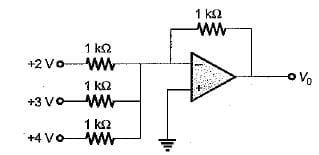Detailed Solution for Test: Operational Amplifier - Question 2

Given circuit represents an inverting summer using op-amp
Here,

R = R1 = R2 = R3 = Rf = 1 kΩ

and

V1 = +2 V, V2 = +3 V, V3 = +4 V

The output voltage is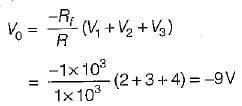Test: Operational Amplifier - Question 3

### For the circuit shown below, the output voltage is given by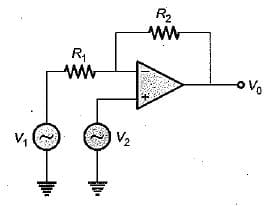Detailed Solution for Test: Operational Amplifier - Question 3

Using superposition theorem, the output voltage is given by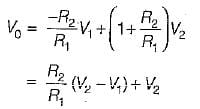Test: Operational Amplifier - Question 4

For an astabie multivibrator shown below,

R1 = 116 KΩ, R2 = 100 KΩ, and ± Vsat = ± 14 V.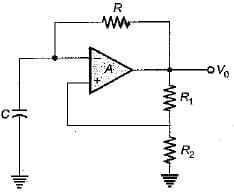The time constant of the circuit to produce an output of frequency 1 kHz will be

Detailed Solution for Test: Operational Amplifier - Question 4

The time period of the given a stablem ultivibrator circuit is given by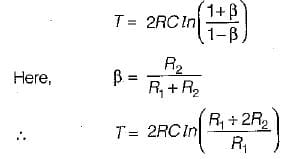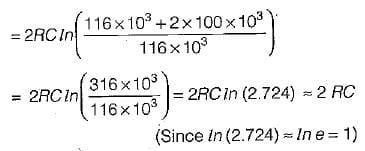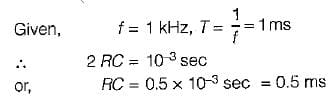Test: Operational Amplifier - Question 5

An ideal operational amplifier has input impedance of

Detailed Solution for Test: Operational Amplifier - Question 5

For an ideal OPAMP, input impedance is infinite while output impedance is zero.

Test: Operational Amplifier - Question 6

For the op-amp circuit shown below, R1 = 1 kΩ and Rf = 10 kΩ. The feedback factor β of this amplifier is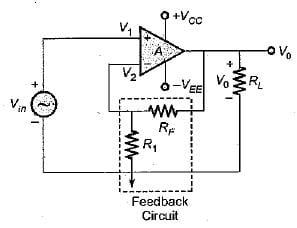Detailed Solution for Test: Operational Amplifier - Question 6

Given op -amp circuit re p re se n ts a c losed -loop non -inverting amplifier. The feed back factor is given by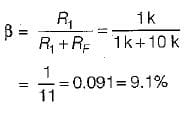Test: Operational Amplifier - Question 7

The order of input resistance in 741 OP-AMP is

Detailed Solution for Test: Operational Amplifier - Question 7

For a practical OPAMP in put impedance is of the order of 106Ω

Test: Operational Amplifier - Question 8

For a given op-amp, CMMR = 105 and differential gain Ad = 105. The common-mode gain of the op-amp is

Detailed Solution for Test: Operational Amplifier - Question 8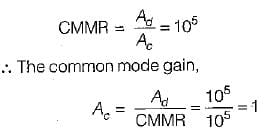Test: Operational Amplifier - Question 9

An inverting amplifier using the 741C must have a fiat response upto 40 kHz. The gain of the amplifier is 10 and slew rate is 0.5 V/μS. The maximum peak-to-peak input signal that can be applied without distorting the output is approximately equal to

Detailed Solution for Test: Operational Amplifier - Question 9

We know that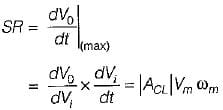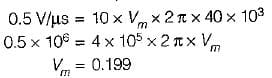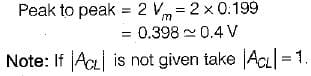Test: Operational Amplifier - Question 10

An idea! operational amplifier has
1. non-zero, off set voltage.
2. characteristics which do not drift with temperature.
3. an infinite voltage gain.
4. a CMMR more than 120 dB.
5. infinite bandwidth.
Of these statements:

Detailed Solution for Test: Operational Amplifier - Question 10

An ideal operational amplifier has

• zero offset voltage
• characteristics which do not drift with temperature
• infinite voltage gain
• infinite CMMR
• infinite bandwidth

Hence, statements 2 , 3 and 5 are only correct.

## Topicwise Question Bank for Electronics Engineering

193 tests
Information about Test: Operational Amplifier Page
In this test you can find the Exam questions for Test: Operational Amplifier solved & explained in the simplest way possible. Besides giving Questions and answers for Test: Operational Amplifier, EduRev gives you an ample number of Online tests for practice

193 tests